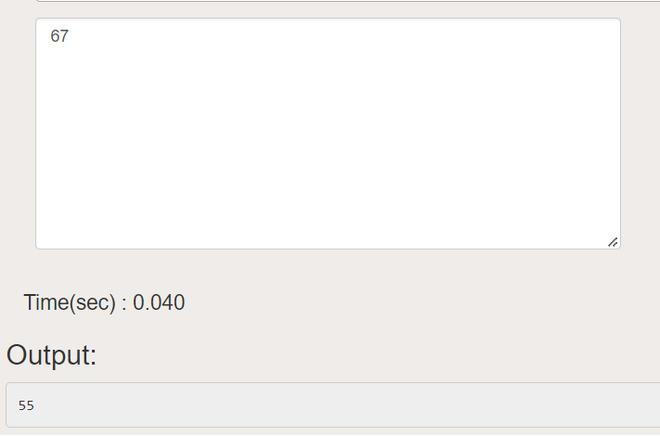# C Program For Octal to Decimal Conversion

• Last Updated : 02 Aug, 2022

Here, we will convert the octal to a decimal using 4 different approaches in C Language:

1. Using Format Specifier
2. Without using pow()
3. Using pow()
4. Using Standard method

Input:

`octal number = 67`

Output:

`decimal number = 55`

## C

 `// C program to demonstrate conversion of``// octal to decimal using format specifier`` ` `#include `` ` `int` `main()``{``    ``int` `n;``    ``scanf``(``"%o"``, &n);``    ``// printing the result``    ``printf``(``"%d"``, n);``    ``return` `0;``}`

Output:Output

## C

 `// C program to demonstrate conversion of``// octal to decimal without using pow()`` ` `#include `` ` `// Function to convert octal to decimal``int` `octalToDecimal(``int` `n)``{``    ``int` `decimalvalue = 0;`` ` `    ``// assigning base value to 1, i.e 8^0``    ``int` `base = 1;`` ` `    ``int` `temp = n;``    ``// while loop executes the statements until the``    ``// condition is false``    ``while` `(temp) {`` ` `        ``// finding the last digit``        ``int` `lastdigit = temp % 10;``        ``temp = temp / 10;`` ` `        ``// Multiplying last digit with appropriate``        ``// base value and adding it to decimalvalue``        ``decimalvalue += lastdigit * base;`` ` `        ``base = base * 8;``    ``}`` ` `    ``return` `decimalvalue;``}`` ` `// Driver program``int` `main()``{``    ``int` `octalnum = 67;`` ` `    ``printf``(``"decimal number is %d"``,``           ``octalToDecimal(octalnum));``}`

Output

`decimal number is 55`

## C

 `// C program to demonstrate conversion of``// octal to decimal using pow()`` ` `#include ``#include `` ` `int` `Octaltodecimal(``int` `octal)``{``    ``int` `decimalnumber = 0, i = 0;`` ` `    ``// while loop executes the statements until the``    ``// condition is false``    ``while` `(octal != 0) {``        ``// calculating equivalent decimal number for the``        ``// given octal number``        ``decimalnumber``            ``= decimalnumber + (octal % 10) * ``pow``(8, i++);``        ``octal = octal / 10;``    ``}``    ``// printing the result``    ``return` `decimalnumber;``}``// driver code``int` `main()``{``    ``int` `octalnumber = 67;``    ``// calling function``    ``printf``(``"decimal number is %d"``,``           ``Octaltodecimal(octalnumber));``    ``return` `0;``}`

Output

`decimal number is 55`

## C

 `// C program to demonstrate conversion of``// octal to decimal without using functions`` ` `#include ``#include ``int` `main()``{`` ` `    ``int` `octalnumber = 67, decimalnumber = 0;``    ``int` `i = 0;``   ` `    ``// while loop executes the statements until the``    ``// condition is false``    ``while` `(octalnumber != 0) {``       ` `        ``// calculating equivalent decimal number for the``        ``// given octal number``        ``decimalnumber = decimalnumber``                        ``+ (octalnumber % 10) * ``pow``(8, i++);``        ``octalnumber = octalnumber / 10;``    ``}``   ` `    ``// printing the result``    ``printf``(``"decimal number is: %d"``, decimalnumber);``    ``return` `0;``}`

Output

`decimal number is: 55`

My Personal Notes arrow_drop_up